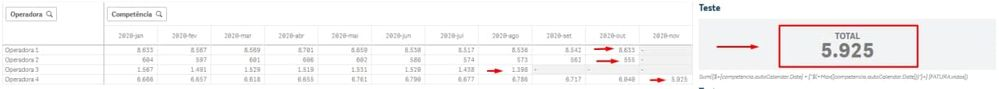# App Development

Announcements
Join us for a live Q&A! September 21, 10 AM ET - Onboarding Fast in Qlik Sense SaaS! REGISTER
cancel
Showing results for
Did you mean:Contributor III

## Sum only the values for the last month of each customer.

Hi! Can someone help me with a tip / example on how to solve this issue:

I need to add in a KPI only the value of the last month found for each operator / customer and the months with data will not always coincide depending on the filter that the user made.

In this example: A customer has data in November, others in September, October and another in August. The formula I'm using takes only the last month of an operator / customer (in the case of the example November, which is the last month with data).Labels (4)

• ### sum

1 Solution

Accepted SolutionsSpecialist III

if the requirement is for each operator, only the ones with the arrows are to be shown, you can use the following expression assuming Operator is your dimension, Date is the yearMonth and SUM(..) is your expression:

= Sum(
Aggr(
If([Date] = Max(TOTAL <[Operator]> Date), SUM([YOUR FIELD HERE]))
, [Date], Operator
)
)

this will return data for each operator where DATE = MAX(DATE) for that operator

3 RepliesSpecialist III

if the requirement is for each operator, only the ones with the arrows are to be shown, you can use the following expression assuming Operator is your dimension, Date is the yearMonth and SUM(..) is your expression:

= Sum(
Aggr(
If([Date] = Max(TOTAL <[Operator]> Date), SUM([YOUR FIELD HERE]))
, [Date], Operator
)
)

this will return data for each operator where DATE = MAX(DATE) for that operatorContributor III
Author

Thank you! He solved the situation perfectly.Specialist III

npTags
Community Browser